We use cookies to enhance your experience on our website. By continuing to use our website, you are agreeing to our use of cookies. You can change your cookie settings at any time. Find out more# Maths education glossary: A to L

Unsure of arrays or perplexed by partitioning? Get to know what your child is learning at school with our guide to common terms and phrases used in schools.

For help with general education terms, take a look at the Education glossary.

For help with literacy terms, take a look at the Grammar and literacy glossary.

A–L | M–Z

A | B | C | D | E | F | G | H | I | J | K | L | M | N | O | P | Q | R | S | T | U | V | W | X | Y | Z

# A

### Acute

An acute angle is an angle that is less than a right angle. It is any angle between 0° and 90°.

### Area

The area of a shape is how much surface it has. Area is measured in square units such as square centimetres (cm²), square metres (m²), and square kilometres (km²).

### Array

Shapes or objects arranged in a rectangle are called an array. Egg boxes or muffin trays are good examples of arrays. Teachers use these to help children to ‘see’ multiplication. For example:

If you have a tray of muffins that is four muffins along and three muffins deep, there will be twelve muffins in total.

### Activity: Multiplication and division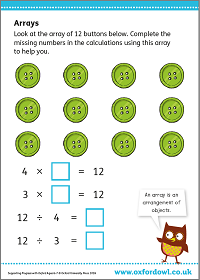Complete the missing numbers in the calculations using this array to help you.

### Average

There are three main types of average: mean, median, and mode.

Mean: To find the mean average of a set of numbers, you need to add the numbers together and then divide the total by the quantity of numbers in the group. For example:

The mean of 6, 3, 9, 3, and 4 is (6 + 3 + 9 + 3 + 4) ÷ 5 = 5.

Median: The middle value of an ordered set of numbers. For example:

The median of 6, 3, 9, 3, and 4 is the middle value of 3, 3, 4, 6, 9 – so the median is 4.

Mode: The number that occurs most often in a set of numbers. For example:

The mode of 6, 3, 9, 3, and 4 is 3, because it occurs most often in the set.

### Video: What are averages?

Find out how to calculate mean, median, and mode averages.

### Axes

This is the plural of axis.

### Axis

1. Many graphs have two axes: a horizontal axis and a vertical axis.2. An axis is a straight line through the middle of a 3D shape. The Earth turns on its axis.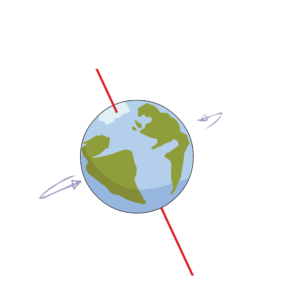# B

### Bar chart

A bar chart (or bar graph) is a graph that uses bars to show information. The bars are all the same thickness and can be horizontal or vertical. The bars usually show two different types of information.### Block graph

A block graph is a graph made up of blocks. The blocks are usually square but are sometimes rectangular.### BODMAS

Mathematical operations can include orders (root and square numbers), division, multiplication, addition, and subtraction. ‘BODMAS’ is a way of remembering the order these operations should be carried out within a calculation: first anything inside brackets, then any squares or roots (orders), then any division and multiplication, and finally any addition and subtraction, working from left to right.

2² × 5 + (10 – 3) = ?
We complete everything in brackets first:
2² × 5 + (10 – 3) = ?
10 – 3 = 7
2² × 5 + 7 = ?
Next, we complete any ‘order’ calculations (squares or roots):
× 5 + 7 = ?
2² = 2 × 2 = 4
4 × 5 + 7 = ?
Next, we complete any division or multiplication:
4 × 5 + 7 = ?
4 × 5 = 20
20 + 7 = ?
Finally, we complete any addition or subtraction:
20 + 7 = ?
20 + 7 = 27
So, 2² × 5 + (10 – 3) = 27

### Activity: Operations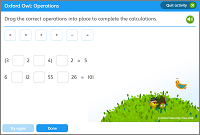Drag the correct operations into place to complete the calculations.

### Video: What is BODMAS?

Find out the right order to solve any calculation.

# C

### Capacity

Capacity is how much something holds. It is usually measured in litres and millilitres.

### Chunking

Chunking is sometimes used as a way of estimating or checking division calculations.

Using multiples of the number that the total has to be divided by breaks down the calculation into sizeable ‘chunks’ that are subtracted from the total. A first step in a calculation such as 615 ÷ 20 could involve subtracting ‘chunks’ of multiples of 20, one step at a time.

615 – 200 – 200 – 200 = 15, so the answer to 615 ÷ 20 must be 30 (multiply 10 × 3 steps), plus a remainder.

### Video: How to do division by chunking

Find out how to do division using the chunking method.

### Circumference

Circumference is the distance all the way around a circle. It is the perimeter of a circle.

### Common factor

A common factor is a number that is a factor of two different numbers.

The common factors of 12 and 18 are 1, 2, 3, and 6 as these are the factors that are common to both numbers.

### Commutative

The commutative law of arithmetic says that you can change the order of the numbers when adding or multiplying and the answer will not change. Knowing this property can help make calculations, especially mental calculations, easier.

Addition and multiplication are both commutative, but subtraction and division are not.
6 + 3 + 4 = 6 + 4 + 3
2 × 6 × 5 = 2 × 5 × 6
7 – 3 ≠ 3 – 7
12 ÷ 4 ≠ 4 ÷ 12

### Composite

1. A composite number is any number with three or more factors. This means it cannot be 0, 1, or a prime number.
2. A composite shape is a 2D shape made from a number of other 2D shapes. The area of composite shapes is often found by breaking up the shape into its composite rectangles or triangles.### Cone

A cone has a flat base that is a circle. The top comes to a point and its sides are curved.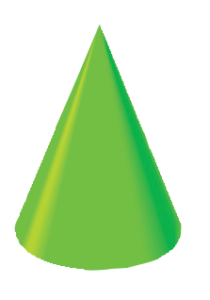### Continuous data

Continuous data is data that can be measured at any point on a scale. It is often shown using a line graph.

The time taken to run a race is continuous data, because it has an infinite number of possibilities within a range. The number of athletes is not continuous data, because it is a finite number that can be counted.

### Coordinates

Coordinates are two numbers or letters that describe a position on maps, graphs and charts. The horizontal coordinate is always written first and the vertical coordinate is always written second.### Cuboid

A cuboid is a 3D shape shaped like a box, with six rectangular faces. A cuboid has six faces, eight vertices and 12 edges.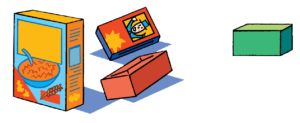### Cylinder

A cylinder is a 3D shape shaped like a roller. It has two flat faces and one curved surface. Cross-sections of a cylinder, parallel to the base, or its flat faces, are all identically sized circles.# D

### Data handling

Using simple lists, tables and graphs to present information.

### Decimals

You use decimal numbers (or decimal notation) when you use hundreds, tens and ones. Decimals are based on ten, multiples of ten, and tenths. A decimal point separates whole numbers from decimal fractions.

124·75 is a decimal number made up of 1 hundred, 2 tens, 4 ones, 7 tenths, and 5 hundredths.

### Denominator

The bottom number of a fraction is called the denominator. The denominator tells us how many equal parts the quantity or shape has been divided into.

In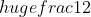, the denominator is 2.

### Diameter

Any straight line from one side of a circle to the other is called a diameter. It must go through the centre.

### Difference

The difference between two numbers is the value of how much one is greater than the other. It can found by subtracting the smallest number from the largest, or by counting on from the smallest to the largest.

The difference between 5 and 2 is 3. 5 – 2 = 3

### Discrete data

Discrete data is data that can be counted. It is shown in distinct, separated groups, such as in bar charts. Data that cannot be counted is called continuous data.

### Division

Division is sharing, grouping, or separating a number into equal parts. Children use lots of different techniques to divide. Take a look at our Division YouTube playlist for detailed guidance.

Short division and long division are both ways of dividing a smaller number into a larger number, one digit at a time, starting on the left. This means working from the largest to the smallest place value, rounding the final digits of the large number at each step. Short division involves ‘carrying’ numbers between place values, whereas long division involves writing down the answer to each step, so is more useful for larger numbers.

Chunking is sometimes used as a way of estimating or checking division calculations. Using multiples of the number that the total has to be divided by breaks down the calculation into sizeable ‘chunks’ that are subtracted from the total. A first step in a calculation such as 615 ÷ 20 could involve subtracting ‘chunks’ of multiples of 20, one step at a time.

### Video: How to do division

Find out how to divide numbers using different methods (YouTube playlist).

# E

### Equilateral

An equilateral triangle has all its sides the same length. Each of its three angles is also the same.### Equivalent

Two things are said to be equivalent to one another if they have the same value, or produce the same effect in use, but have different forms.

£1 is equivalent (in value) to 100 pence.

½ is equivalent to 0.5.

# F

### Factor

A factor is a whole number that another number can divide into without any remainders.

1, 2, 3, 4, 6, and 12 are all factors of 12.

### Factor pairs

A factor pair is a pair of numbers that are multiplied together to get a particular number. For example:

3 and 2 are a factor pair of 6, because 3 × 2 = 6.

### Financial education

An emphasis on numeracy skills, using money and working with percentages.

### Formal Written Methods

The standard procedures used for calculations that cannot easily be solved mentally. These include:

• Column addition and column subtraction: The process of writing numbers one above the other and then calculating each column in turn, beginning with the units column and working upwards in place value.
• Short multiplication and long multiplication: The process of multiplying two numbers by writing the numbers vertically, then multiplying the larger number by each digit of the smaller number, starting from the units column and then working upwards in place value. Short multiplication is suitable for multiplying a large number by a single-digit number, whereas long multiplication involves writing down the answers to each step, so is more useful for multiplying two large numbers.
• Short division and long division: The process of dividing a smaller number into a larger number, one digit at a time, starting on the left. This means working from the largest to the smallest place value, effectively rounding the final digits of the large number at each step. Short division involves ‘carrying’ numbers between place values, whereas long division involves writing down the answer to each step, so is more useful for dividing by larger numbers.

### Video playlist: How to do division

Find out how to divide numbers using different methods.

### Formula

A formula is a rule that tells you how to work something out based on certain values. A formula can be given in words or using letters and symbols.

The formula for finding the area of a rectangle is “area = length × width”.

### Fraction

A fraction is an equal part of a whole, like(one half) or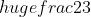(two thirds).

If you hadof a cherry pie, the pie would be cut into two equal pieces, and you would get one piece.
If you hadof a cherry pie, the pie would be cut into three equal pieces, and you would get two pieces.

Numerator: The top number of a fraction is called the numerator. The numerator tells us how many equal parts there are.

In, the numerator is 1.

Unit fraction: A unit fraction is a fraction which has 1 as its numerator.is a unit fraction, because the numerator is 1.

Non-unit fraction: A non-unit fraction is a fraction where the numerator is greater than 1.is a non-unit fraction, because the numerator is 2.

Improper fraction: An improper fraction is a fraction with a numerator bigger than its denominator. This means the fraction is greater than 1.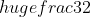is an improper fraction, because its numerator (3) is bigger than its denominator (2).

Mixed number: A mixed number is another way of showing an improper fraction, by mixing a whole number and a proper fraction.

For example: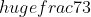(an improper fraction) can also be represented as 2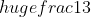(a mixed number).

### Activity: Paper fractions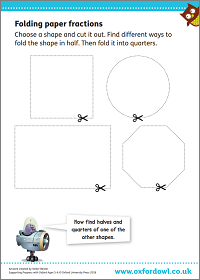Find halves and quarters of different shapes.

### Video: What are fractions?

Find out all about numerators, denominators, improper fractions, mixed numbers, and simplifying fractions.

# G

### Grid method

Schools sometimes use the grid method as a way of visualising multiplication, as part of formal calculations. For example:

To work out 65 × 74 using the grid method, you set the numbers out in a grid:You then multiply the numbers separately and add them together to find the total:

70 × 60 = 4200

70 × 5 = 350

4 × 60 = 240

4 × 5 = 20

4200 + 350 + 240 + 20 = 4810

# I

### Improper fraction

An improper fraction is a fraction with a numerator bigger than its denominator. This means the fraction is greater than 1.is an improper fraction, because its numerator (3) is bigger than its denominator (2).

### Inverse operations

We say that addition and subtraction are inverse operations. This means that we can use one operation to ‘undo’ the other. For example, you can undo adding 5 by taking away 5. Multiplication and division are also inverse operations. If you multiply a number by 10, you can undo this by dividing by 10.

Children are taught to use inverse operations to check their answers to a question. For example:

If they think 15 + 35 = 50, they can check this by subtracting 35 from 50 to see if they get 15.

### Irregular

Irregular polygons do not have all their sides the same length. They have different size angles.

### Isosceles

An isosceles triangle has two sides that are the same length. Two angles are also equal.# K

### Kite

A kite is a four-sided polygon. It has two pairs of adjacent sides that are the same length.# L

### Line of symmetry

See division.

A line of symmetry divides a shape in half. One half is the reflection of the other half. The line of symmetry is the same as a mirror line. Some shapes have no lines of symmetry while others have one or more.### Long division

See division.

A–L | M–Z

A | B | C | D | E | F | G | H | I | J | K | L | M | N | O | P | Q | R | S | T | U | V | W | X | Y | Z